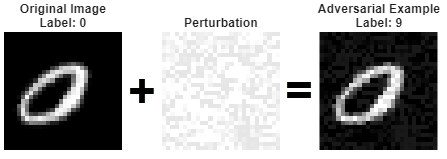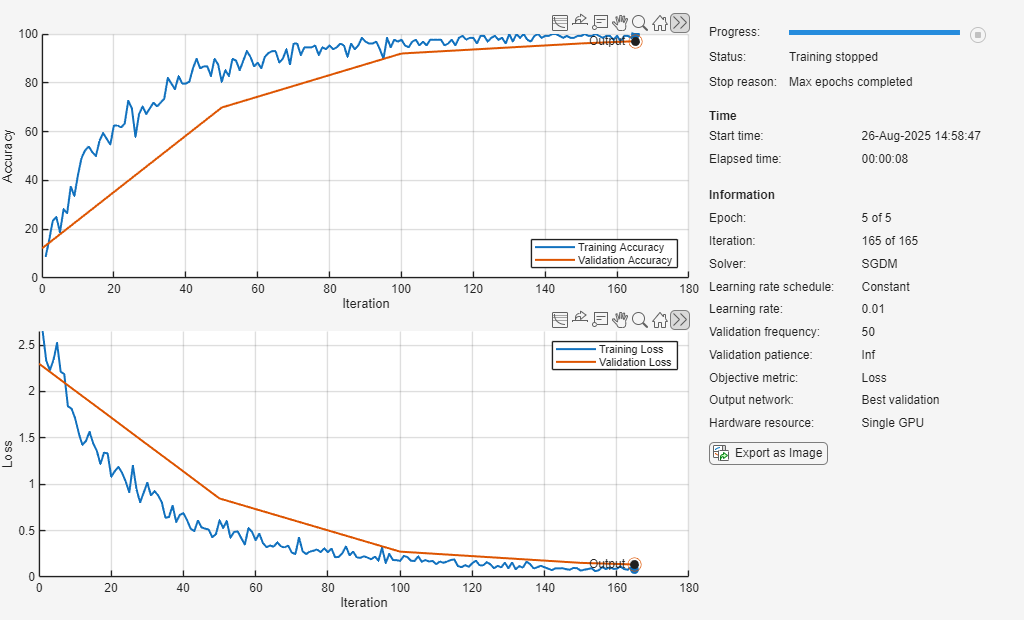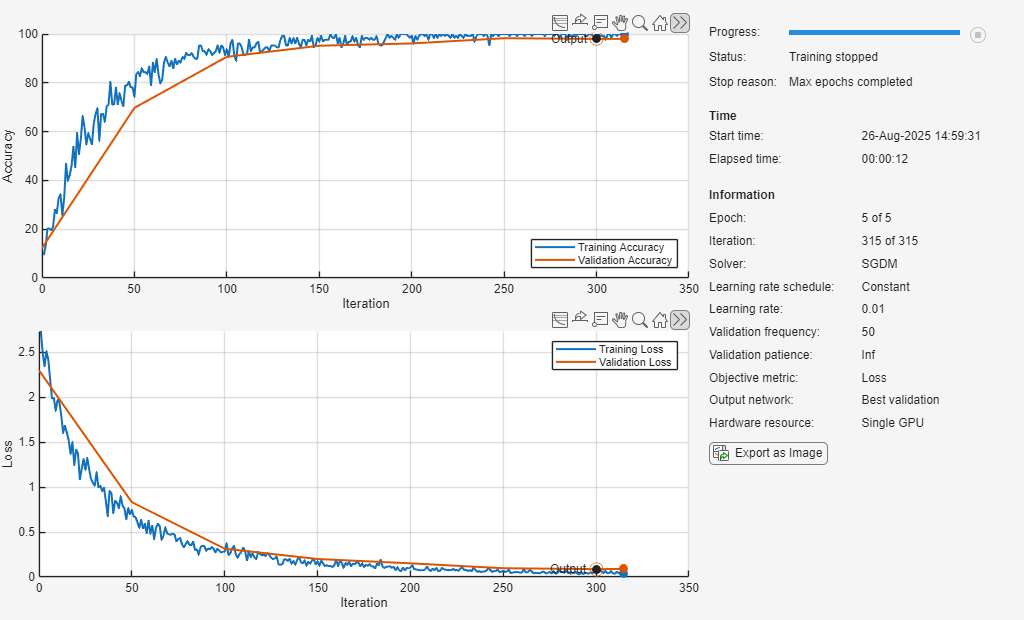# Train Image Classification Network Robust to Adversarial Examples

This example shows how to train a neural network that is robust to adversarial examples using fast gradient sign method (FGSM) adversarial training.

Neural networks can be susceptible to a phenomenon known as adversarial examples , where very small changes to an input can cause it to be misclassified. These changes are often imperceptible to humans.Techniques for creating adversarial examples include the FGSM  and the basic iterative method (BIM) , also known as projected gradient descent . These techniques can significantly degrade the accuracy of a network.

You can use adversarial training  to train networks that are robust to adversarial examples. This example shows how to:

1. Train an image classification network.

2. Investigate network robustness by generating adversarial examples.

3. Train an image classification network that is robust to adversarial examples.

The `digitTrain4DArrayData` function loads images of handwritten digits and their digit labels. Create an `arrayDatastore` object for the images and the labels, and then use the `combine` function to make a single datastore containing all the training data.

```rng default [XTrain,TTrain] = digitTrain4DArrayData; dsXTrain = arrayDatastore(XTrain,'IterationDimension',4); dsTTrain = arrayDatastore(TTrain); dsTrain = combine(dsXTrain,dsTTrain);```

Extract the class names.

`classes = categories(TTrain);`

### Construct Network Architecture

Define an image classification network.

```layers = [ imageInputLayer([28 28 1],'Normalization','none','Name','input') convolution2dLayer(3,32,'Padding',1,'Name','conv1') reluLayer('Name','relu1') convolution2dLayer(3,64,'Padding',1,'Name','conv3') reluLayer('Name','relu3') maxPooling2dLayer(2,'Stride',2,'Name','pool') fullyConnectedLayer(10,'Name','fc2') softmaxLayer('Name','softmax')]; lgraph = layerGraph(layers);```

Create a `dlnetwork` object from the layer graph.

`dlnet = dlnetwork(lgraph);`

Create the function `modelGradients`, listed at the end of the example, that takes as input a `dlnetwork` object and a mini-batch of input data with corresponding labels and returns the gradients of the loss with respect to the learnable parameters in the network and the corresponding loss.

### Train Network

Train the network using a custom training loop.

Specify the training options. Train for 30 epochs with a mini-batch size of 100 and a learning rate of 0.01.

```numEpochs = 30; miniBatchSize = 100; learnRate = 0.01; executionEnvironment = "auto";```

Create a `minibatchqueue` object that processes and manages mini-batches of images during training. For each mini-batch:

• Use the custom mini-batch preprocessing function `preprocessMiniBatch` (defined at the end of this example) to convert the labels to one-hot encoded variables.

• Format the image data with the dimension labels `'SSCB'` (spatial, spatial, channel, batch).

• Train on a GPU if one is available. By default, the `minibatchqueue` object converts each output to a `gpuArray` if a GPU is available. Using a GPU requires Parallel Computing Toolbox™ and a supported GPU device. For information on supported devices, see GPU Support by Release (Parallel Computing Toolbox).

```mbq = minibatchqueue(dsTrain, ... 'MiniBatchSize',miniBatchSize,... 'MiniBatchFcn',@preprocessMiniBatch,... 'MiniBatchFormat',{'SSCB',''});```

Initialize the training progress plot.

```figure lineLossTrain = animatedline('Color',[0.85 0.325 0.098]); ylim([0 inf]) xlabel("Iteration") ylabel("Loss") grid on```

Initialize the velocity parameter for the SGDM solver.

`velocity = [];`

Train the network using a custom training loop. For each epoch, shuffle the data and loop over mini-batches of data. For each mini-batch:

• Evaluate the model gradients, state, and loss using the `dlfeval` and `modelGradients` functions and update the network state.

• Update the network parameters using the `sgdmupdate` function.

• Display the training progress.

```iteration = 0; start = tic; % Loop over epochs. for epoch = 1:numEpochs % Shuffle data. shuffle(mbq) % Loop over mini-batches. while hasdata(mbq) iteration = iteration +1; % Read mini-batch of data. [dlX,dlT] = next(mbq); % If training on a GPU, then convert data to gpuArray. if (executionEnvironment == "auto" && canUseGPU) || executionEnvironment == "gpu" dlX = gpuArray(dlX); dlT = gpuArray(dlT); end % Evaluate the model gradients, state, and loss. [gradients,state,loss] = dlfeval(@modelGradients,dlnet,dlX,dlT); dlnet.State = state; % Update the network parameters using the SGDM optimizer. [dlnet,velocity] = sgdmupdate(dlnet,gradients,velocity,learnRate); % Display the training progress. D = duration(0,0,toc(start),'Format','hh:mm:ss'); addpoints(lineLossTrain,iteration,loss) title("Epoch: " + epoch + ", Elapsed: " + string(D)) drawnow end end```### Test Network

Test the classification accuracy of the network by evaluating network predictions on a test data set.

Create a `minibatchqueue` object containing the test data.

```[XTest,TTest] = digitTest4DArrayData; dsXTest = arrayDatastore(XTest,'IterationDimension',4); dsTTest = arrayDatastore(TTest); dsTest = combine(dsXTest,dsTTest); mbqTest = minibatchqueue(dsTest, ... 'MiniBatchSize',miniBatchSize, ... 'MiniBatchFcn',@preprocessMiniBatch, ... 'MiniBatchFormat','SSCB');```

Predict the classes of the test data using the trained network and the `modelPredictions` function defined at the end of this example.

```YPred = modelPredictions(dlnet,mbqTest,classes); acc = mean(YPred == TTest)```
```acc = 0.9866 ```

The network accuracy is very high.

### Test Network with Adversarial Inputs

Apply adversarial perturbations to the input images and see how doing so affects the network accuracy.

You can generate adversarial examples using techniques such as FGSM and BIM. FGSM is a simple technique that takes a single step in the direction of the gradient ${\nabla }_{X}L\left(X,T\right)$ of the loss function $L$, with respect to the image $X$ you want to find an adversarial example for, and the class label $T$. The adversarial example is calculated as

${\mathit{X}}_{\mathrm{adv}}=\mathit{X}+ϵ.\mathrm{sign}\left({\nabla }_{\mathit{X}}\mathit{L}\left(\mathit{X},\mathit{T}\right)\right)$.

Parameter $ϵ$ controls how different the adversarial examples look from the original images. In this example, the values of the pixels are between 0 and 1, so an $ϵ$ value of 0.1 alters each individual pixel value by up to 10% of the range. The value of $ϵ$ depends on the image scale. For example, if your image is instead between 0 and 255, you need to multiply this value by 255.

BIM is a simple improvement to FGSM which applies FGSM over multiple iterations and applies a threshold. After each iteration, the BIM clips the perturbation to ensure the magnitude does not exceed $ϵ$. This method can yield adversarial examples with less distortion than FGSM. For more information about generating adversarial examples, see Generate Untargeted and Targeted Adversarial Examples for Image Classification.

Create adversarial examples using the BIM. Set `epsilon` to 0.1.

`epsilon = 0.1;`

For the BIM, the size of the perturbation is controlled by parameter $\alpha$ representing the step size in each iteration. This is as the BIM usually takes many, smaller, FGSM steps in the direction of the gradient.

Define the step size `alpha` and the number of iterations.

```alpha = 0.01; numAdvIter = 20;```

Use the `adversarialExamples` function (defined at the end of this example) to compute adversarial examples using the BIM on the test data set. This function also returns the new predictions for the adversarial images.

```reset(mbqTest) [XAdv,YPredAdv] = adversarialExamples(dlnet,mbqTest,epsilon,alpha,numAdvIter,classes);```

Compute the accuracy of the network on the adversarial example data.

`accAdversarial = mean(YPredAdv == TTest)`
```accAdversarial = 0.0114 ```

Plot the results.

`visualizePredictions(XAdv,YPredAdv,TTest);`You can see that the accuracy is severely degraded by the BIM, even though the image perturbation is hardly visible.

### Train Robust Network

You can train a network to be robust against adversarial examples. One popular method is adversarial training. Adversarial training involves applying adversarial perturbations to the training data during the training process  .

FGSM adversarial training is a fast and effective technique for training a network to be robust to adversarial examples. The FGSM is similar to the BIM, but it takes a single larger step in the direction of the gradient to generate an adversarial image.

Adversarial training involves applying the FGSM technique to each mini-batch of training data. However, for the training to be effective, these criteria must apply:

• The FGSM training method must use a randomly initialized perturbation instead of a perturbation that is initialized to zero.

• For the network to be robust to perturbations of size $ϵ$, perform FGSM training with a value slightly larger than $ϵ$. For this example, during adversarial training, you perturb the images using step size $\alpha =1.25ϵ$.

Train a new network with FGSM adversarial training. Start by using the same untrained network architecture as in the original network.

`dlnetRobust = dlnetwork(lgraph); `

Define the adversarial training parameters. Set the number of iterations to 1, as the FGSM is equivalent to the BIM with a single iteration. Randomly initialize the perturbation and perturb the images using `alpha`.

```numIter = 1; initialization = "random"; alpha = 1.25*epsilon;```

Initialize the training progress plot.

```figure lineLossRobustTrain = animatedline('Color',[0.85 0.325 0.098]); ylim([0 inf]) xlabel("Iteration") ylabel("Loss") grid on```

Train the robust network using a custom training loop and the same training options as previously defined. This loop is the same as in the previous custom training, but with added adversarial perturbation.

```velocity = []; iteration = 0; start = tic; % Loop over epochs. for epoch = 1:numEpochs % Shuffle data. shuffle(mbq) % Loop over mini-batches. while hasdata(mbq) iteration = iteration + 1; % Read mini-batch of data. [dlX,dlT] = next(mbq); % If training on a GPU, then convert data to gpuArray. if (executionEnvironment == "auto" && canUseGPU) || executionEnvironment == "gpu" dlX = gpuArray(dlX); dlT = gpuArray(dlT); end % Apply adversarial perturbations to the data. dlX = basicIterativeMethod(dlnetRobust,dlX,dlT,alpha,epsilon, ... numIter,initialization); % Evaluate the model gradients, state, and loss. [gradients,state,loss] = dlfeval(@modelGradients,dlnetRobust,dlX,dlT); dlnet.State = state; % Update the network parameters using the SGDM optimizer. [dlnetRobust,velocity] = sgdmupdate(dlnetRobust,gradients,velocity,learnRate); % Display the training progress. D = duration(0,0,toc(start),'Format','hh:mm:ss'); addpoints(lineLossRobustTrain,iteration,loss) title("Epoch: " + epoch + ", Elapsed: " + string(D)) drawnow end end```### Test Robust Network

Calculate the accuracy of the robust network on the digits test data. The accuracy of the robust network can be slightly lower than the nonrobust network on the standard data.

```reset(mbqTest) YPred = modelPredictions(dlnetRobust,mbqTest,classes); accRobust = mean(YPred == TTest)```
```accRobust = 0.9972 ```

```reset(mbqTest) [XAdv,YPredAdv] = adversarialExamples(dlnetRobust,mbqTest,epsilon,alpha,numAdvIter,classes); accRobustAdv = mean(YPredAdv == TTest)```
```accRobustAdv = 0.7558 ```

The adversarial accuracy of the robust network is much better than that of the original network.

### Supporting Functions

The `modelGradients` function takes as input a `dlnetwork` object `dlnet` and a mini-batch of input data `dlX` with corresponding labels T and returns the gradients of the loss with respect to the learnable parameters in `dlnet`, the network state, and the loss. To compute the gradients automatically, use the `dlgradient` function.

```function [gradients,state,loss] = modelGradients(dlnet,dlX,T) [dlYPred,state] = forward(dlnet,dlX); loss = crossentropy(dlYPred,T); gradients = dlgradient(loss,dlnet.Learnables); loss = double(gather(extractdata(loss))); end```

The `modelGradientsInput` function takes as input a `dlnetwork` object `dlnet` and a mini-batch of input data `dlX` with corresponding labels T and returns the gradients of the loss with respect to the input data `dlX`.

```function gradient = modelGradientsInput(dlnet,dlX,T) T = squeeze(T); T = dlarray(T,'CB'); [dlYPred] = forward(dlnet,dlX); loss = crossentropy(dlYPred,T); gradient = dlgradient(loss,dlX); end```

#### Mini-Batch Preprocessing Function

The `preprocessMiniBatch` function preprocesses a mini-batch of predictors and labels using the following steps:

1. Extract the image data from the incoming cell array and concatenate into a four-dimensional array.

2. Extract the label data from the incoming cell array and concatenate into a categorical array along the second dimension.

3. One-hot encode the categorical labels into numeric arrays. Encoding into the first dimension produces an encoded array that matches the shape of the network output.

```function [X,T] = preprocessMiniBatch(XCell,TCell) % Concatenate. X = cat(4,XCell{1:end}); X = single(X); % Extract label data from the cell and concatenate. T = cat(2,TCell{1:end}); % One-hot encode labels. T = onehotencode(T,1); end```

#### Model Predictions Function

The `modelPredictions` function takes as input a `dlnetwork` object `dlnet`, a `minibatchqueue` of input data `mbq`, and the network classes, and computes the model predictions by iterating over all data in the `minibatchqueue` object. The function uses the `onehotdecode` function to find the predicted class with the highest score.

```function predictions = modelPredictions(dlnet,mbq,classes) predictions = []; while hasdata(mbq) dlXTest = next(mbq); dlYPred = predict(dlnet,dlXTest); YPred = onehotdecode(dlYPred,classes,1)'; predictions = [predictions; YPred]; end end```

Generate adversarial examples for a `minibatchqueue` object using the basic iterative method (BIM) and predict the class of the adversarial examples using the trained network `dlnet`.

```function [XAdv,predictions] = adversarialExamples(dlnet,mbq,epsilon,alpha,numIter,classes) XAdv = {}; predictions = []; iteration = 0; % Generate adversarial images for each mini-batch. while hasdata(mbq) iteration = iteration +1; [dlX,dlT] = next(mbq); initialization = "zero"; % Generate adversarial images. XAdvMBQ = basicIterativeMethod(dlnet,dlX,dlT,alpha,epsilon, ... numIter,initialization); % Predict the class of the adversarial images. dlYPred = predict(dlnet,XAdvMBQ); YPred = onehotdecode(dlYPred,classes,1)'; XAdv{iteration} = XAdvMBQ; predictions = [predictions; YPred]; end % Concatenate. XAdv = cat(4,XAdv{:}); end```

#### Basic Iterative Method Function

Generate adversarial examples using the basic iterative method (BIM). This method runs for multiple iterations with a threshold at the end of each iteration to ensure that the entries do not exceed `epsilon`. When `numIter` is set to 1, this is equivalent to using the fast gradient sign method (FGSM).

```function XAdv = basicIterativeMethod(dlnet,dlX,dlT,alpha,epsilon,numIter,initialization) % Initialize the perturbation. if initialization == "zero" delta = zeros(size(dlX),'like',dlX); else delta = epsilon*(2*rand(size(dlX),'like',dlX) - 1); end for i = 1:numIter % Apply adversarial perturbations to the data. gradient = dlfeval(@modelGradientsInput,dlnet,dlX+delta,dlT); delta = delta + alpha*sign(gradient); delta(delta > epsilon) = epsilon; delta(delta < -epsilon) = -epsilon; end XAdv = dlX + delta; end```

#### Visualize Prediction Results Function

Visualize images along with their predicted classes. Correct predictions use green text. Incorrect predictions use red text.

```function visualizePredictions(XTest,YPred,TTest) figure height = 4; width = 4; numImages = height*width; % Select random images from the data. indices = randperm(size(XTest,4),numImages); XTest = extractdata(XTest); XTest = XTest(:,:,:,indices); YPred = YPred(indices); TTest = TTest(indices); % Plot images with the predicted label. for i = 1:(numImages) subplot(height,width,i) imshow(XTest(:,:,:,i)) % If the prediction is correct, use green. If the prediction is false, % use red. if YPred(i) == TTest(i) color = "\color{green}"; else color = "\color{red}"; end title("Prediction: " + color + string(YPred(i))) end end```

### References

 Szegedy, Christian, Wojciech Zaremba, Ilya Sutskever, Joan Bruna, Dumitru Erhan, Ian Goodfellow, and Rob Fergus. “Intriguing Properties of Neural Networks.” Preprint, submitted February 19, 2014. https://arxiv.org/abs/1312.6199.

 Goodfellow, Ian J., Jonathon Shlens, and Christian Szegedy. “Explaining and Harnessing Adversarial Examples.” Preprint, submitted March 20, 2015. https://arxiv.org/abs/1412.6572.

 Kurakin, Alexey, Ian Goodfellow, and Samy Bengio. “Adversarial Examples in the Physical World.” Preprint, submitted February 10, 2017. https://arxiv.org/abs/1607.02533.

 Madry, Aleksander, Aleksandar Makelov, Ludwig Schmidt, Dimitris Tsipras, and Adrian Vladu. “Towards Deep Learning Models Resistant to Adversarial Attacks.” Preprint, submitted September 4, 2019. https://arxiv.org/abs/1706.06083.

 Wong, Eric, Leslie Rice, and J. Zico Kolter. “Fast Is Better than Free: Revisiting Adversarial Training.” Preprint, submitted January 12, 2020. https://arxiv.org/abs/2001.03994.